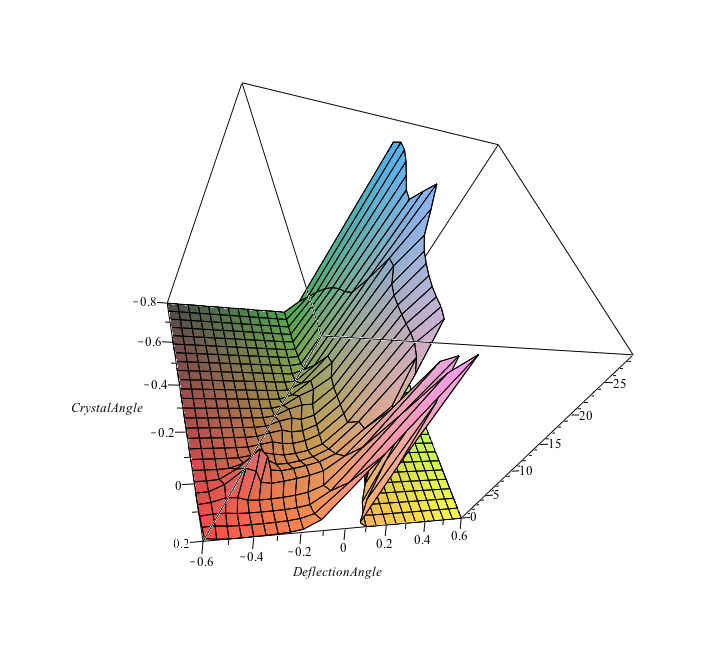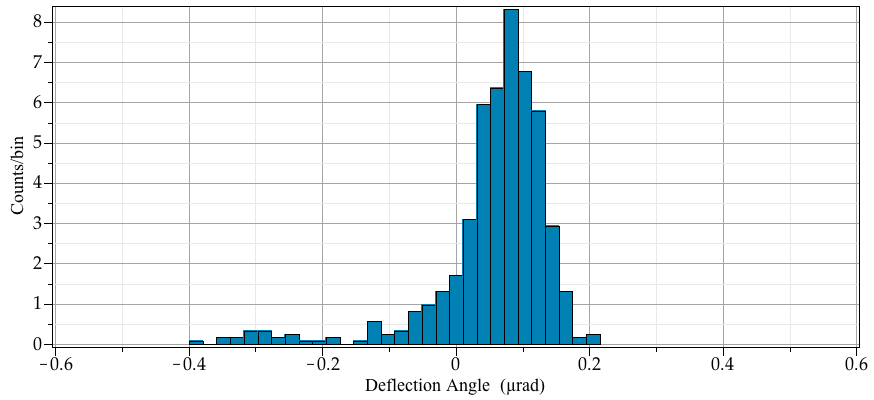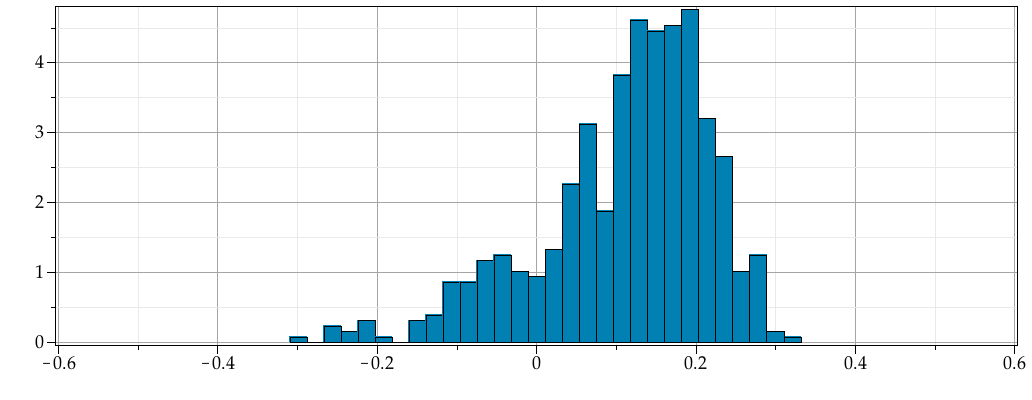:

## Random numbers

Maple

Erik Postma from Maplesoft has recently posted a Maple application going through some of the ways how to produce random numbers in Maple: http://www.maplesoft.com/applications/view.aspx?SID=153662&P=TC-4444

Doing a fair bit of work involving random numbers (i.e. simulations) myself, I was naturally drawn to it. The document is a nice extension of the relevant help pages, making practical use of some of the procs in RandomTools easier and clearer.

One particular issue I recently ran into, however, is not covered. I needed a random generator for a particular custom pdf (not one of the already recognized pdfs) that happens to be 3-dimensional. And I needed to do this >twice<, with the second generation using the result of the first one as a parameter. the problem is not unlike the last example in the help page for Statistics:-Sample.

Here is what I did:

The pdf looks graphically like this (and note that in this plot it is not normalized):

plot3d(Triangle63:-Trianglef(CrystalAngle,DeflectionAngle),\
CrystalAngle=-0.8..0.2,DeflectionAngle=-0.6..0.6,projection=0.7);The first set of random numbers is generated by setting CrystalAngle to a fixed number (-0.1 in this case), normalizing the pdf to the integral over DeflectionAngle and then generating the random numbers. Note that evaluation of the integral is relatively time-consuming (seconds).

trianglepdf:=(CrystalAngle,DeflectionAngle) ->\
Triangle63:-Trianglef(CrystalAngle,DeflectionAngle)/\
'evalf(Int(Triangle63:-Trianglef(CrystalAngle,Da),Da=-0.6..0.6,method = _d01akc))':
triangle0pdf:=(t) -> trianglepdf(-0.1,t):
Cr1:=Distribution(PDF=triangle0pdf);
Y:=RandomVariable(Cr1);

samples:=Sample(Y,[1..600],method=[envelope,range=-0.6..0.6]);This looks as it should; and the random number generation is reasonably fast once the initial setup has been done (i.e. the time used scales only relatively weakly with the count of random numbers generated.

The issue now is the second stage, where for each new random number, the number generated above is a new input parameter for CrystalAngle. So I can no longer define the pdf once since the pdf is different for each number generated.

The pedestrian way to do this is like the following:

samples2:=Vector(1..numelems(samples),datatype=float):
CrystalOffset:=0.3;

for ii from 1 to numelems(samples) do
triangleiipdf:=(t) -> (trianglepdf(samples[ii]-~CrystalOffset,t)); # subtract (VR peak -> 0)
Crii:=Distribution(PDF=triangleiipdf);
samples2[ii]:=evalf(Sample(RandomVariable(Crii),1,method=[envelope,range=-0.6..0.6])+CrystalOffset);
end do:

This works, but only sort-of. First, it is very slow, since the whole sertup happens for each number generated (Sample()). Secondly, it tends to hang at a certain number of steps through the loop. The value of ii where it hangs is arbitrary and changes with the other parameters and CrystalOffset. It is however consistent for identical runs.

The last example in help for Statistics:-Sample would indicate that one needs to setup the pdf as a function of t as well as the parameter, call Distribution() only once and then assign the value to the parameter and call Sample() to get the random number(s) drawn from the pdf with the parameter being set to the wanted value. While this works in the example which uses a built-in pdf, I find that I cannot make it work with my pdf. Either the parameter gets ignored (i.e. stuck at the first value) or the thing runs just as slowly as my procedure above.

Ultimately I programmed my own random generator for an arbitrary pdf which, while not as efficient as Maple's built-in generator, does produce the expected set of random numbers for the 2nd path in a reasonable time. It is a simple-minded envelope-rejection method, that works fo reasonably well-behaved pdfs:

Rarbit:=proc(pdf,xmin,xmax,pdfmax)
local dx:=xmax-xmin;
local x1,x2;
x1:=RandomTools[MersenneTwister]:-GenerateFloat64()*dx+xmin; # pick location on x axis
x2:=RandomTools[MersenneTwister]:-GenerateFloat64()*pdfmax; # y axis, probability of returning x1

while (x2>evalf(pdf(x1))) do # if above pdf, reject
x1:=RandomTools[MersenneTwister]:-GenerateFloat64()*dx+xmin; # new x-axis value
x2:=RandomTools[MersenneTwister]:-GenerateFloat64()*pdfmax; # check value
end do; # eventually we'll succeed and return one.

x1;
end proc;

Using this routine I get my second and final set of randome:

for ii from 1 to numelems(samples) do
newpdf:=(DefAng) -> Triangle63:-Trianglef(samples[ii]-CrystalOffset,DefAng);
samples2[ii]:=Random:-Rarbit(newpdf,-0.6,0.6,28);
DocumentTools:-SetProperty(ProgressBar,'value',ii,refresh);
end do:
CrystalOffset := 0.3
Histogram(samples2+~samples,view=[-0.6..0.6,default]);I would be interested in Erik's comment on this.

Mac Dude﻿